# 题目描述（困难难度）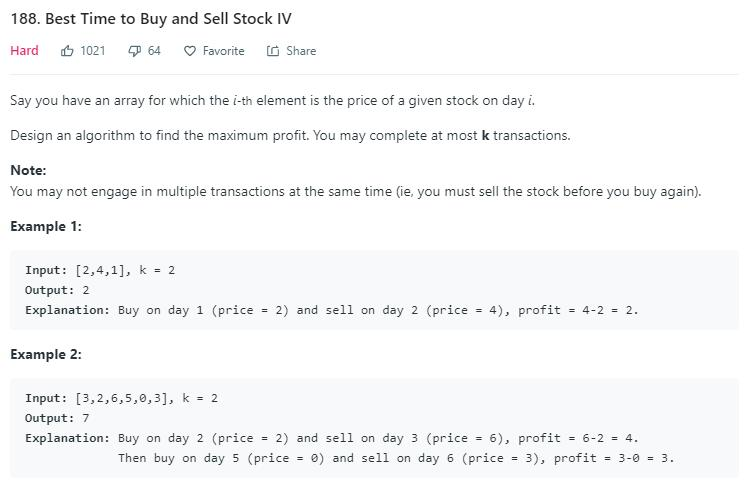# 解法一

123 题 要求最多买卖两次，最终优化的出的代码如下。

public int maxProfit(int[] prices) {
if (prices.length == 0) {
return 0;
}
int K = 2;
int[] dp = new int[K + 1];
int min[] = new int[K + 1];
for (int i = 1; i <= K; i++) {
min[i] = prices;
}
for (int i = 1; i < prices.length; i++) {
for (int k = 1; k <= K; k++) {
min[k] = Math.min(prices[i] - dp[k - 1], min[k]);
dp[k] = Math.max(dp[k], prices[i] - min[k]);
}
}
return dp[K];
}


public int maxProfit(int k, int[] prices) {
if (prices.length == 0) {
return 0;
}
int K = k;
int[] dp = new int[K + 1];
int min[] = new int[K + 1];
for (int i = 1; i <= K; i++) {
min[i] = prices;
}
for (int i = 1; i < prices.length; i++) {
for (int kk = 1; kk <= K; kk++) {
min[kk] = Math.min(prices[i] - dp[kk - 1], min[kk]);
dp[kk] = Math.max(dp[kk], prices[i] - min[kk]);
}
}
return dp[K];
}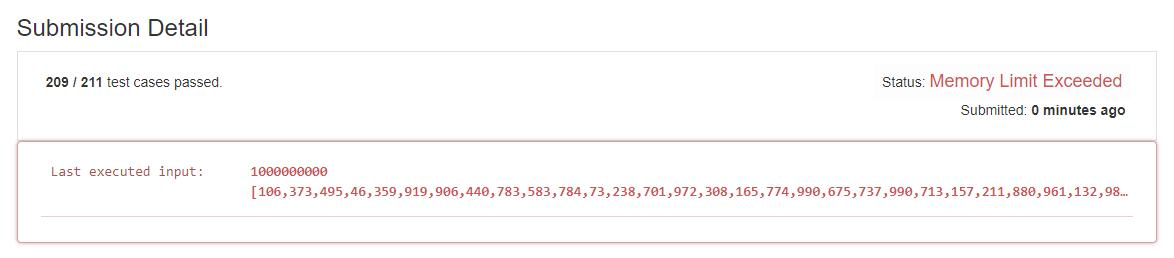public int maxProfit(int k, int[] prices) {
if (prices.length == 0) {
return 0;
}
int K = Math.min(k, prices.length / 2);
int[] dp = new int[K + 1];
int min[] = new int[K + 1];
for (int i = 1; i <= K; i++) {
min[i] = prices;
}
for (int i = 1; i < prices.length; i++) {
for (int kk = 1; kk <= K; kk++) {
min[kk] = Math.min(prices[i] - dp[kk - 1], min[kk]);
dp[kk] = Math.max(dp[kk], prices[i] - min[kk]);
}
}
return dp[K];
}


public int maxProfit(int k, int[] prices) {
if (prices.length == 0) {
return 0;
}
//K 看做任意次，转到 122 题
if (k >= prices.length / 2) {
return maxProfit(prices);
}
int K = k;
int[] dp = new int[K + 1];
int min[] = new int[K + 1];
for (int i = 1; i <= K; i++) {
min[i] = prices;
}
for (int i = 1; i < prices.length; i++) {
for (int kk = 1; kk <= K; kk++) {
min[kk] = Math.min(prices[i] - dp[kk - 1], min[kk]);
dp[kk] = Math.max(dp[kk], prices[i] - min[kk]);
}
}
return dp[K];
}

//122 题代码
public int maxProfit(int[] prices) {
int profit = 0;
for (int i = 1; i < prices.length; i++) {
int sub = prices[i] - prices[i - 1];
if (sub > 0) {
profit += sub;
}
}
return profit;
}


# 解法二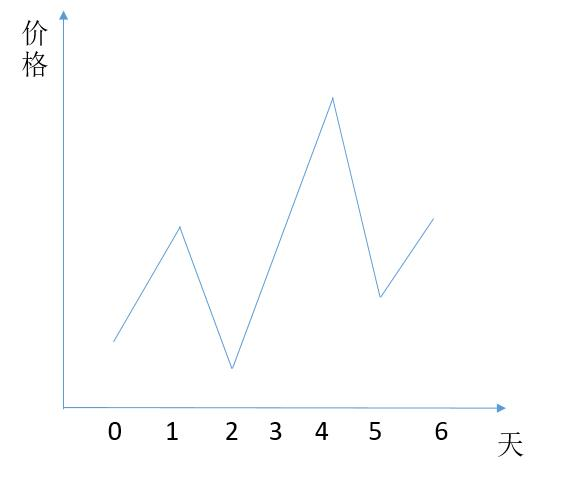//定义一个结构，存储一次交易的买入卖出时间
class Transaction {
int valley;
int peek;

Transaction(int v, int p) {
valley = v;
peek = p;
}
}

public int maxProfit(int k, int[] prices) {
if(k == 0){
return 0;
}
Stack<Transaction> stack = new Stack<>();
List<Integer> profit = new ArrayList<>();
int v;
int p = -1;
int n = prices.length;
while (true) {
v = p + 1;
//寻找波谷
while (v + 1 < n && prices[v] > prices[v + 1]) {
v++;
}
p = v;
//寻找波峰
while (p + 1 < n && prices[p] <= prices[p + 1]) {
p++;
}

//到达最后，结束寻找
if (p == v) {
break;
}

//将这次的波谷、波峰存入
stack.push(new Transaction(v, p));
}

//遍历所有的买入、卖出，计算其收益
while (!stack.isEmpty()) {
Transaction pop = stack.pop();
}
int ret = 0;
//如果能够进行的交易数 K 大于我们存的交易数，就把所有收益累加
if (k >= profit.size()) {
for (int i = 0; i < profit.size(); i++) {
ret += profit.get(i);
}
} else {
//将收益从大到小排序
Collections.sort(profit, new Comparator<Integer>() {
@Override
public int compare(Integer n1, Integer n2) {
return n2 - n1;
}
});

//选取前 k 个
for (int i = 0; i < k; i++) {
ret += profit.get(i);
}
}
return ret;
}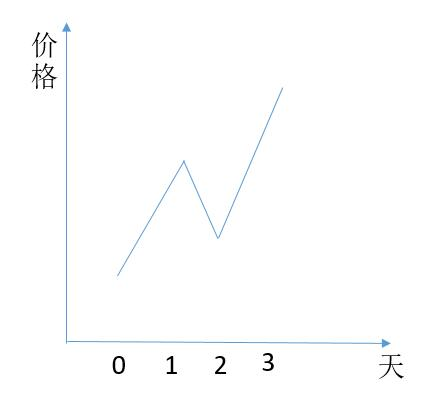while (!stack.isEmpty() && prices[p] >= prices[stack.peek().peek && prices[v] >= prices[stack.peek().valley]) {
Transaction pop = stack.pop();
//加入 prices[p1] - prices[v2] 的收益
//买入点更新为前一次的买入点
v = pop.valley;
}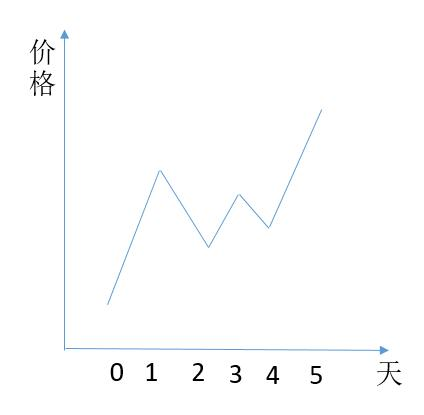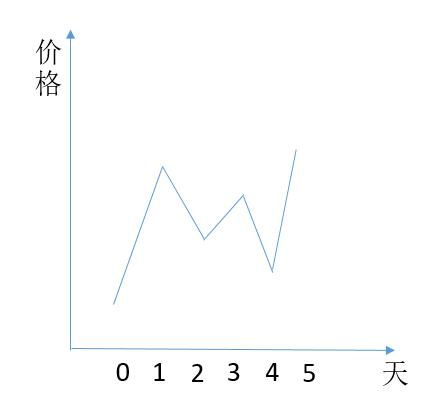//当前的买入点比栈顶的低
while (!stack.isEmpty() && prices[v] <= prices[stack.peek().valley]) {
Transaction pop = stack.pop();
}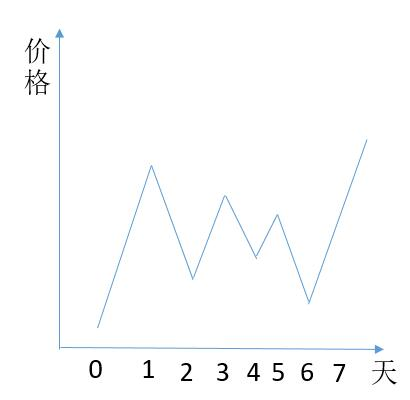(6,7)来的时候，要把 (4,5)(2,3) 依次出栈。

class Transaction {
int valley;
int peek;

Transaction(int v, int p) {
valley = v;
peek = p;
}
}

public int maxProfit(int k, int[] prices) {
if(k == 0){
return 0;
}
Stack<Transaction> stack = new Stack<>();
List<Integer> profit = new ArrayList<>();
int v;
int p = -1;
int n = prices.length;
while (true) {
v = p + 1;
while (v + 1 < n && prices[v] > prices[v + 1]) {
v++;
}
p = v;
while (p + 1 < n && prices[p] <= prices[p + 1]) {
p++;
}

if (p == v) {
break;
}

//新的交易的买入点更低，要把栈顶的元素出栈
while (!stack.isEmpty() && prices[v] <= prices[stack.peek().valley]) {
Transaction pop = stack.pop();
}

//当前交易和栈顶交易是否能合并
while (!stack.isEmpty() && prices[p] >= prices[stack.peek().peek]) {
Transaction pop = stack.pop();
v = pop.valley;
}

stack.push(new Transaction(v, p));
}

while (!stack.isEmpty()) {
Transaction pop = stack.pop();
}
int ret = 0;
if (k >= profit.size()) {
for (int i = 0; i < profit.size(); i++) {
ret += profit.get(i);
}
} else {
Collections.sort(profit, new Comparator<Integer>() {
@Override
public int compare(Integer n1, Integer n2) {
return n2 - n1;
}
});

for (int i = 0; i < k; i++) {
ret += profit.get(i);
}
}
return ret;
}


//相当于最小堆，队列头始终队列中是最小的元素
PriorityQueue<Integer> queue = new PriorityQueue<Integer>();

for (int i = 0; i < profit.size(); i++) {
if (i < k) {
} else {
int peek = queue.peek();
//当前收益大于栈顶元素，将栈顶元素弹出，然后将当前元素加入队列
if (profit.get(i) > peek) {
queue.poll();
}
}

}

while (!queue.isEmpty()) {
ret += queue.poll();
}